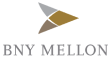New update is available. Click here to update.
Topics

Moderate0/80
Average time to solve is 35m+7 more companies

## Problem statement

A ninja needs to complete ‘n’ tasks. Each task is represented by an uppercase letter of the English alphabet. Different letters are assigned to different tasks. A ninja can complete tasks in any order. He takes one unit of time to complete one task. For each unit of time, he could complete either one task or just be idle.

Ninja easily gets bored by doing the same task again. So he decided to keep at least ‘t’ units of time between any two same tasks.

You are given a string ‘tasks’ consisting of ‘n’ uppercase letters of the English alphabet, representing the tasks ninja need to complete, and an integer ‘t’ representing the least units of time between any two same tasks. Find out the minimum total units of time ninja will take to complete all ‘n’ tasks.

Detailed explanation ( Input/output format, Notes, Images )
Constraints :
``````1 <= T <= 50
1 <= n <= 10^4
1 <= t <= 100

Where ‘T’ is the number of test cases and ‘n’ is the number of tasks and ‘t’ is the least units of time between any two same tasks.

Time Limit : 1 sec
``````
Sample Input 1 :
``````2
6 2
AAABBB
1 5
A
``````
Sample Output 1 :
``````8
1
``````
Explanation of the Sample Input1 :
``````Test case 1 :

One possible way to complete tasks is :-

Doing task ‘A’ in the first unit of time.

Doing task ‘B’ in the second unit of time because ninjas want to have at least 2 units of time between the same task, so we could not choose A.

Be Idle in the third unit of time  because ninjas want to have at least 2 units of time between the same task, so we could not choose any one of  A or B.

Doing task ‘A’ in the fourth unit of time.

Doing task ‘B’ in the fifth unit of time.

Be Idle in the sixth unit of time.

Doing task ‘A’ in the seventh unit of time.

Doing task ‘B’ in the third unit of time.

Note that, there are at least 2 units of time between any two same tasks. We can show that there is no way to complete the task in less than 8 units of time.

Test case 2 :

There is only one task that can be completed in one unit of time.
``````
Sample Input 2 :
``````2
5 0
AAABB
2 10
ZZ
``````
Sample Output 2 :
``````5
12
``````
Explanation of the Sample Input 2 :
``````Test case 1 :

Since ‘t’ = 0, a ninja can complete tasks without becoming idle at any unit of time.

Test case 2 :

A ninja can complete the first task ‘Z’ in the first unit of time then becomes idle for the next 10 seconds and then complete the second task ‘Z’ in the twelfth unit of time.
``````Console